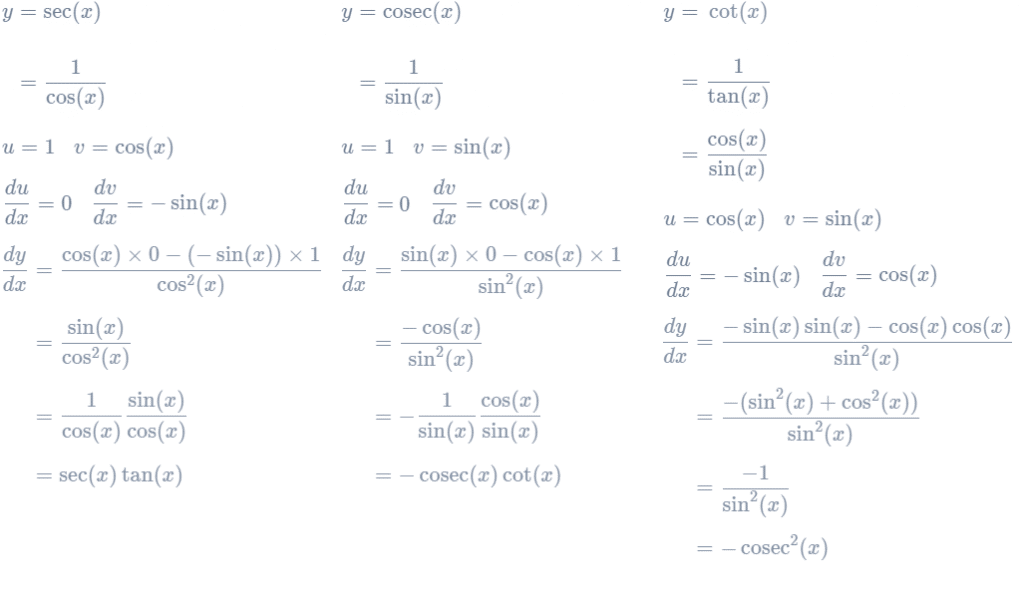# More Differentiation

A LevelAQAEdexcelOCR

## More Differentiation

This section contains further uses of the chain, product and quotient rules, combining them with other things you have learned so far such as trigonometry and stationary points.

Make sure you are happy with the following topics before continuing.

A LevelAQAEdexcelOCR

## Derivatives of sec, cosec and cot

Use the quotient rule to find the derivatives of $\sec(x)$, $\cosec(x)$ and $\cot(x)$.

Recall: Quotient rule

$\dfrac{dy}{dx}=\dfrac{v\dfrac{du}{dx}-u\dfrac{dv}{dx}}{v^{2}}$A LevelAQAEdexcelOCR

## Using the Chain, Product and Quotient Rule With Special Functions

Now that we know how to differentiate every exponential function and every trigonometric function, we can use them along with our rules. Remember when to use each rule:

• Function of a function – chain rule
• Multiplying two functions together – product rule
• Fraction made of functions – quotient rule

Example: Find the derivative of $\cosec(4x^{3})$

This is a function of a function, so use the chain rule.

$\dfrac{dy}{dx}=\dfrac{dy}{dt}\dfrac{dt}{dx}$

Take $t=4x^{3}$.

Then $y=\cosec(t)$

$\dfrac{dy}{dt}=-\cosec(t)\cot(t)$

$\dfrac{dt}{dx}=12x^{2}$

Hence: $\dfrac{dy}{dx}=-\cosec(t)\cot(t)12x^{2}$

Put $x$ back in:

$\dfrac{dy}{dx}=-12x^{2}\cosec(4x^{3})\cot(4x^{3})$

A LevelAQAEdexcelOCR

## Using the Rules Twice

Sometimes you might need to use the chain, product or quotient rule twice to get a derivative.

Example: Differentiate $2x^{2}\cos(e^{x})$

This is a product of two functions so use product rule.

$\dfrac{dy}{dx}=u\dfrac{dv}{dx}+v\dfrac{du}{dx}$

$u=2x^{2}\;\;\;v=\cos(e^{x})$

$\dfrac{du}{dx}=4x$

To find $\dfrac{dv}{dx}$ we need to use the chain rule.

$\dfrac{dv}{dx}=\dfrac{dv}{dt}\dfrac{dt}{dx}$

Take $t=e^{x}$

Then $v=\cos(t)$

$\dfrac{dv}{dt}=-\sin(t)$

$\dfrac{dt}{dx}=e^{x}$

Hence: $\dfrac{dv}{dx}=-\sin(t)e^{x}$

Put $x$ back in:

$\dfrac{dv}{dx}=-e^{x}\sin(e^{x})$

Now we can complete our product rule.

$\dfrac{dy}{dx}=-2x^{2}e^{x}\sin(e^{x})+4x\cos(e^{x})$

A LevelAQAEdexcelOCR

## Using the Rules to find the Second Derivative

We already know that second derivatives can be very useful when it comes to classifying stationary points. However, starting with expressions like these, finding the second derivative can get very complicated.

Example: $y=e^{3x^{2}}$. Find $\dfrac{d^{2}y}{dx^{2}}$.

To differentiate once, we need the chain rule, because this is a function within a function.

$\dfrac{dy}{dx}=\dfrac{dy}{dt}\dfrac{dt}{dx}$

Take $t=3x^{2}$

Then $y=e^{t}$

$\dfrac{dy}{dt}=e^{t}$

$\dfrac{dt}{dx}=6x$

Hence: $\dfrac{dy}{dx}=6xe^{t}$

Put $x$ back in:

$\dfrac{dy}{dx}=6xe^{3x^{2}}$

To differentiate a second time, we notice we have two functions multiplied together, so must use the product rule.

$\dfrac{d^{2}y}{dx^{2}}=u\dfrac{dv}{dx}+v\dfrac{du}{dx}$

$u=6x\;\;\;v=e^{3x^{2}}$

$\dfrac{du}{dx}=6$

$\dfrac{dv}{dx}$ requires the chain rule, but it is identical to the differentiation we just did above.

$\dfrac{dv}{dx}=6xe^{3x^{2}}$

Hence:

\begin{aligned}\dfrac{d^{2}y}{dx^{2}}&=6x\times6xe^{3x^{2}}+6e^{3x^{2}}\\[1.2em]&=36x^{2}e^{3x^{2}}+6e^{3x^{2}}\\[1.2em]&=6(6x^{2}+1)e^{3x^{2}}\end{aligned}

A LevelAQAEdexcelOCR

## More Differentiation Example Questions

a) i) $\sec(x)\tan(x)$

ii) $-\cosec(x)\cot(x)$

iii) $-\cosec^{2}(x)$

b) $y=\sec(\cot(x))$

This is a function of a function, so use chain rule.

$\dfrac{dy}{dx}=\dfrac{dy}{dt}\dfrac{dt}{dx}$

Take $t=\cot(x)$

Then $y=\sec(t)$

$\dfrac{dy}{dt}=\sec(t)\tan(t)$

$\dfrac{dt}{dx}=-\cosec^{2}(x)$

$\dfrac{dy}{dx}=-\sec(t)\tan(t)\cosec^{2}(x)$

Put $x$ back in

$\dfrac{dy}{dx}=-\sec(\cot(x))\tan(\cot(x))\cosec^{2}(x)$

This is a product of two functions, so use product rule.

$\dfrac{dy}{dx}=u\dfrac{dv}{dx}+v\dfrac{du}{dx}$

$u=\sin(3x^{2})\;\;\;v=\ln(x)$

$\dfrac{dv}{dx}=\dfrac{1}{x}$

For $\dfrac{du}{dx}$ we need the chain rule because we have a function within a function.

$\dfrac{du}{dx}=\dfrac{du}{dt}\dfrac{dt}{dx}$

Take $t=3x^{2}$

Then $y=\sin(t)$

$\dfrac{du}{dt}=\cos(t)$

$\dfrac{dt}{dx}=6x$

$\dfrac{du}{dx}=6x\cos(t)$

Put $x$ back in

$\dfrac{du}{dx}=6x\cos(3x^{2})$

Put into product rule:

$\dfrac{dy}{dx}=\dfrac{\sin(3x^{2})}{x}+6x\ln(x)\cos(3x^{2})$
$y=9e^{\frac{1}{2}x+\cos(x)}$

First, find the derivative using the chain rule.

$\dfrac{dy}{dx}=9\left(\dfrac{1}{2}-\sin(x)\right)e^{\frac{1}{2}x+cos(x)}$

Stationary points are where this is equal to $0$.

$9\left(\dfrac{1}{2}-\sin(x)\right)e^{\frac{1}{2}x+cos(x)}=0$

Exponentials can never be equal to $0$, so:

$9\left(\dfrac{1}{2}-\sin(x)\right)=0$

$\dfrac{1}{2}-\sin(x)=0$

$\sin(x)=\dfrac{1}{2}$

$x=\dfrac{\pi}{6},\dfrac{5\pi}{6}$

Find $y$-coordinate of stationary points:

$y=9e^{\frac{1}{2}\times\frac{\pi}{6}+\cos(\frac{\pi}{6})}$

$y=9e^{\frac{\pi}{12}+\frac{\sqrt{3}}{2}}$

$y=9e^{\frac{1}{2}\times\frac{5\pi}{6}+\cos(\frac{5\pi}{6})}$

$y=9e^{\frac{5\pi}{12}-\frac{\sqrt{3}}{2}}$

Stationary points are:

$\left(\dfrac{\pi}{6},9e^{\frac{\pi}{12}+\frac{\sqrt{3}}{2}}\right),\left(\dfrac{5\pi}{6},9e^{\frac{5\pi}{12}-\frac{\sqrt{3}}{2}}\right)$

Next, differentiate again using the product rule.

$u=9\left(\dfrac{1}{2}-\sin(x)\right)$

$v=e^{\frac{1}{2}x+\cos(x)}$

$\dfrac{du}{dx}=-9\cos(x)$

$\dfrac{dv}{dx}=\left(\dfrac{1}{2}-\sin(x)\right)e^{\frac{1}2{x}+\cos(x)}$

$\dfrac{d^{2}y}{dx^{2}}=9\left(\left(\dfrac{1}{2}-\sin(x)\right)^{2}-\cos(x)\right)e^{\frac{1}{2}x-\cos(x)}$

Before substituting in $x=\dfrac{\pi}{6}$ and $x=\dfrac{5\pi}{6}$, we should first notice that the exponential part of the expression is positive for any $x$, so we need not keep it when trying to determine the sign of $\dfrac{d^{2}y}{dx^{2}}$.

Want to determine the sign of $9\left(\left(\dfrac{1}{2}-\sin(x)\right)^{2}-\cos(x)\right)$

Secondly, we notice that the $x$ values came from $\dfrac{1}{2}-\sin(x)=0$, so this part will remain $0$ when substituting the $x$ values in. We can also get rid of the factor of $9$ without changing the sign.

This means that all we need to do is find the sign of $-\cos(x)$.

$-\cos\left(\dfrac{\pi}{6}\right)=-\dfrac{\sqrt{3}}{2}<0$ so this is a maximum.

$-\cos\left(\dfrac{5\pi}{6}\right)=\dfrac{\sqrt{3}}{2}>0$ so this is a minimum.

Hence:

$\left(\dfrac{\pi}{6},9e^{\frac{\pi}{12}+\frac{\sqrt{3}}{2}}\right)$ is a maximum.

$\left(\dfrac{5\pi}{6},9e^{\frac{5\pi}{12}-\frac{\sqrt{3}}{2}}\right)$ is a maximum.

A Level

A Level

## You May Also Like...### MME Learning Portal

Online exams, practice questions and revision videos for every GCSE level 9-1 topic! No fees, no trial period, just totally free access to the UK’s best GCSE maths revision platform.

£0.00

A Level

A Level

A Level